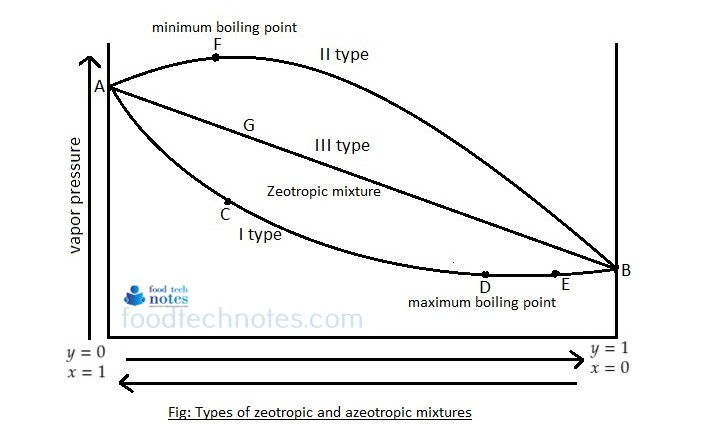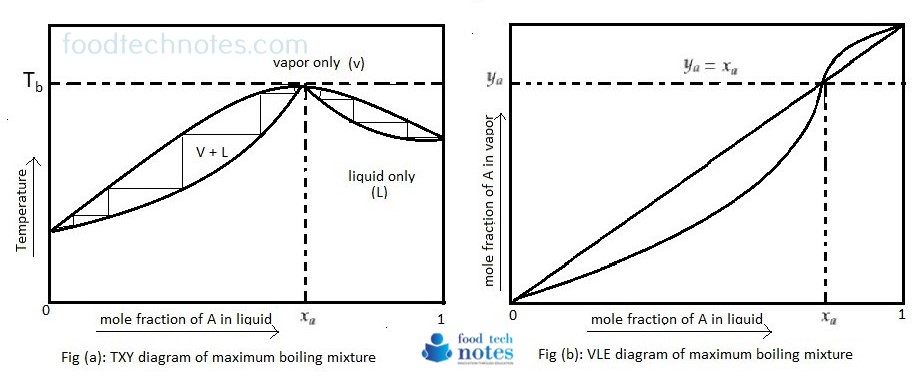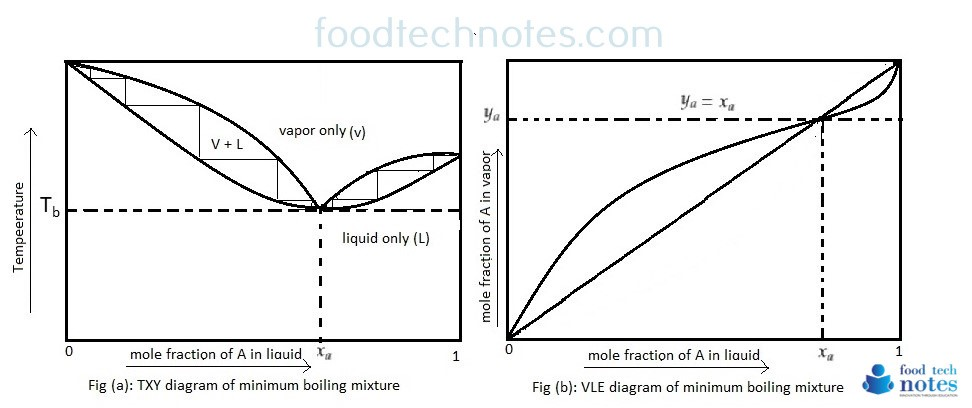# Types Of Mixtures (for distillation)

Miscible liquid mixtures are of three types which can be revealed by plotting the vapor pressure against mole fraction of component.1. First type of mixture:

The Vapor pressure curve exhibit minimum area. If we take mixture which has excess of ‘x’ (more volatile compound), we are somewhere at ‘C’ on the curve. On distillation, vapor will contain excess of ‘x’ and thus the remaining mixture will get richer in ‘y’. Finally we reach the point ‘D’ where vapor pressure is minimum and thus the boiling point is maximum. Here the mixture will distill unchanged in composition.

Similarly, if we take a mixture having greater proportion of ‘y’ (point E) on distillation, ‘y’ will pass over leaving the residue richer in ‘x’. In this way the point ‘D’ is again reached when the mixture is distilled unchanged.

It is obvious that complete separation of this type of solution is impossible. This type of solution which distills unchanged at constant temperature and shows a maximum boiling are called maximum boiling point azeotropic mixtures. For example; mixture of chloroform and acetone. Such mixtures may be represented in boiling point of equilibrium diagram as follows.In figure ’a’, the boiling point Tb corresponding to the concentration xa is the highest temperature reached by any mixture of these substances and higher than the temperature of either pure components. In figure ‘b’, the equilibrium curve touches each other and have common horizontal tangent. The composition of vapor produced from an azeotrope is the same as that of vapor. An azeotrope that can be boiled away at constant pressure without change in concentration is either liquid or vapor. Since under these condition the temperature can’t vary, azeotropes are also called constant boiling mixtures.

Azeotrope cannot be separated by constant pressure distillation into components. If total pressure is changed, the azeotropic components is usually shifted and the principle can be utilized to obtain separation under pressure or vacuum that cannot be obtained at atmospheric pressure. Addition of third component that destroys azeotrope may be employed to obtain the same result.

2. Second type of mixture:

These are also called minimum boiling point azeoptropic solutions. The equilibrium boiling point and equilibrium diagram are shown below.In figure ‘a’, the vapor pressure curve records a maximum at ‘Tb’. At this point, the mixture has the highest vapor pressure and therefore the lowest boiling point. Example; benzene and ethanol. Ethanol and water also give the similar result.

3. Third type of mixture:

This type of mixture always lies between the vapor pressure of pure component and thus the vapor pressure component curve is straight line and is called zeotropic mixture. They can be completely separated by fractional distillation.